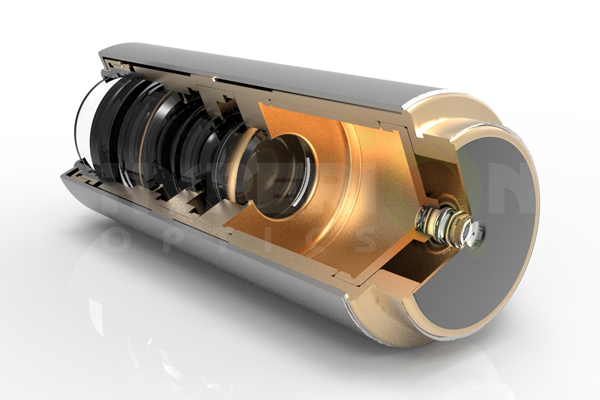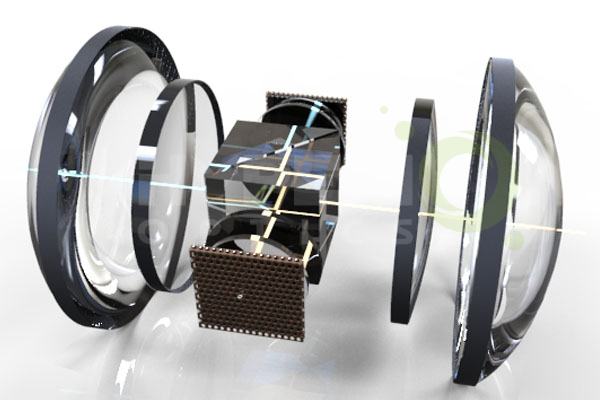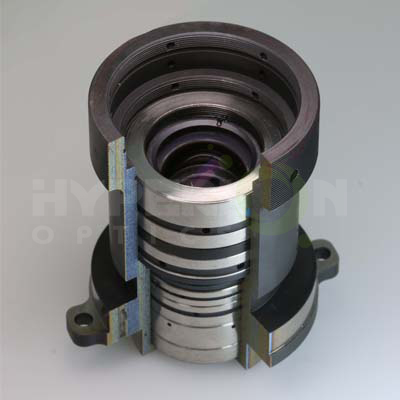﻿ Optical System Design - Hyperion Optics

# Optical System Design

The use of any kind of optical instruments and the conditions of use will certainly make demands on its optical system. Therefore, we must understand its requirements to the optical system before we carry out the optical design. These requirements are summarized in the following aspects.I. The Fundamental Characteristic of Optical System
The fundamental characteristic of optical system is: Numerical aperture or relative aperture; linear field or field angle; systems magnification or focal length. In addition, there are some related characteristics, such as, the size and location of pupil, working distance and conjugate distance.

II. The Systems Overall Dimension
The overall dimensions of the system, i.e., the horizontal and vertical dimensions of the system. In the design of complex optical systems. It is imperative that designers shall correctly define the compatibility of optical element groups pupil.

III. Imaging Quality
Imaging quality requirements related to the use of optical systems. Classified by the applications use, there are different imaging quality requirements on different optical systems. For the telescope system and the general microscope, it is necessary to have a good image quality only in the central field of view. But for the photographic lens, the good quality requirement is needed to meet in the whole field of view.

IV. The Use Conditions of Instrument
When we propose make the demand on optical system the function of the, we must consider the possibility of feasibility of both technical and physical aspects realization. Such as biological magnification should fit into the range of be among 500NA≤Г≤1000NA, when we develop telescope system, in terms of consider the telescope visual magnification, resolution limit of both telescoping system and human eyes must be taken into consideration.

The Design Process of Optical System
Optical system design is the process of determining a variety of data on the basis of use conditions and meets the application imaging quality use requirements, which is, determining the performance parameters of the optical system, the overall dimensions and the structure of the optical element group, etc. Therefore, the optical design process can be divided into 4 stages: the dimension calculation, the initial structure calculation, the aberration correction and balance, and the image quality evaluation.
I. The Overall Size Calculation

At this stage , we need to draw up the design principle of optical system, determine the basic optical properties, which will meet a given technical requirement, that is, the magnification or focal length, lineal field or field angle, numerical aperture or relative aperture, conjugate distance, after working distance of diaphragm position and external dimension etc.. Therefore, this stage is often called the overall dimension calculation. Generally, we calculate the overall dimensions according to perfect optical system theory and calculation formula . And in the process, the mechanical structure and electrical system must be considered in order to prevent the occurs of infeasible structure. The determination of each performance must be reasonable, if it is too high,  the design results will be wasted, if it is too low, the design will not meet the requirements, so this step is need to be considered carefully.

II. The Calculation and Selection of the Initial Structure
The following two methods are usually used to determine the initial structure:
1． Solve the primary aberration theory according to the initial structure.
The method of solving the initial structure is based on the fundamental characteristics calculated from the overall dimensions, and the primary aberration theory is used to solve the initial structure which meet the image quality requirements.
2．Solve the initial structure from the existing document
This is a practical and easy to achieve methods. So it is widely used by many optical designers. But it requires designers to have a deep understanding of the theory of optics, and has a wealth of experience in design. Only this, can he pick out a simple and demanding initial structure from a wide range of structures. The choice of the initial structure is the basis of the lens design. A bad initial structure, no matter how good the automatic design process and experienced designers can not make the design successful.

III. The Aberration Correction and Balance
After the initial structure is selected, the optical path is calculated on the computer with the optical calculation program, and all the aberration and the aberration curves are calculated. According to the analysis of aberration data, it is possible to find out which aberration is the main influence on the imaging quality of optical system. And then, we can find out the modified methods and correct the aberration. Image difference analysis and balance is an iterative process until the image quality requirements are met.

IV. The Image Quality Evaluation
The imaging quality of the optical system is related to the size of the aberration. The purpose of the optical design is to correct the aberration of the optical system. However, it is not possible for any optical system to adjust all the aberrations to zero, and the existence of residual aberration is inevitable. Therefore, the optical designer must have the knowledge of the tolerance value of the optical system’s residual and the aberration tolerance, so as to judge the imaging quality of the optical system according to the residual aberration. There are many methods to evaluate the imaging quality of the optical system. We will briefly introduce the aberration evelation method

1. Rayleigh’s Judgment
The largest wave aberration between the actual wave surface and the ideal wave surface is not more than 1/4 wavelength. It is a more rigorous method to evaluate image quality, which is suitable for small aberration system, such as telescope, micro-objective and so on.

2. Resolution
Resolution refers to the optical system’s ability in terms of distinguishing the object’s details . When the center of the diffraction image of a point coincides with the first dark ring of another point, it is precisely the boundary of the two points that can be separated.

3. Diapoint
When a lot of light emitted by a point go through the optical system, the aberration will cause the intersection between the light and the image plane not focus on the same point, and forming a dispersed graphics within a certain range, called the diapoint. It is usually used as a practical and effective dispersion spot with a concentration of more than 30% points or a circle of light. The reciprocal of its diameter is the number distinguished by the system. It is generally used to evaluate the large aberration system.

4.  Optical Transfer Function
This method is based on the theory that the object is composed of the spectruma with variety of frequencies, that is, the object's brightness distribution function expands as the Fourier series or Fourier integral. The optical system is regarded as a linear invariant system, so that the image of an object through an optical system can be regarded as the transmission of a series of linear systems with different frequencies. Transmission is characterized by the same frequency, but declining contrast, the moving phase to a certain frequency. The decrease of contrast and the change of phase vary with frequency, and the relation between them is called optical transfer function. Because the optical transfer function is related to the image difference, it can be used to evaluate the imaging quality of optical system. It is objective, reliable, and easy to be calculated and measured. It is not only be used to evaluate the results of optical design, but also to control all aspects of the optical system design, optical lens inspection and the process of general design .### The Design Difference Among Different Kinds of Lens

I. Camera lens
The optical properties of the camera lens can be represented by three parameters: the focal length of the camera lens F', the relative aperture D/f' and the field angle (2 Omega). In fact, in terms of 135 cameras, its standard frame has been determined as 24mm X 36mm, the diagonal length is 2D=43.266. From the following table we can see that there is a relationship between the focal length of the camera lens and the field angle f': tgω'=D/f'
In this formula: 2D - diagonal length of frame;
F'- focal length of lens.

Another important optical feature of the camera lens is the relative aperture. It represents the ability of the lens to pass through the light, expressed in D/f'. It is defined as the ratio of the lens aperture diameter (also known as pupil diameter) D and lens focal length F'. The reciprocal of the relative aperture is known as the aperture factor or aperture of the lens, also known as the F, that is, F=f'/D. When the focal length F'is fixed, the F is inversely proportional to the pupil diameter D. Because the area of the light is proportional to the square of the D, the larger the area of the light, the greater the luminous flux of the lens. Therefore, when the number of apertures is in the smallest value, the hole is in its largest value, luminous flux is also the largest. With the increase of the number of apertures, the light hole becomes smaller and the luminous flux decreases. If you do not consider the impact of different lens with transmittance differences, no matter how long the lens focal length is, regardless of how far the lens aperture diameter is, as long as the aperture values are the same, they have the same luminous flux. Compared with the camera lens, F is a very important parameter, the smaller the F value , the wider the scope of the lens.
Compared with the visual optical system, camera lens have a large relative aperture and large field of view, therefore, in order to see the clear and object plane similar image on the whole image plane, almost all the seven kinds of aberration need to be corrected. The resolution of the photographic objective lens is a comprehensive reflection of the relative aperture and aberration residue. After the relative aperture is determined, the optimal error correction scheme is developed, which can meet the requirements and is easy to realize. For convenience, Disperse spot radius is often used to measure the size of the aberration, and the optical transfer function is used to evaluate the image quality.

In recent years, the rising digital camera lens is similar with the traditional camera lens in the aspects of design evaluation and properties, the main difference is:
1 relative aperture is larger than traditional camera.
2 short focal length causes the depth of field increases. According to the size of the field angle, we can calculate the equivalent of the traditional camera lens focal length value F '=43.266/ (2*tg).
3 high resolution, according to the size of the PIXEL in the photoelectric device, the general digital lens optical design will achieve 1/ (line) pairs.

II. The projection lens
The projection lens refers to the illuminated object will form a bright and clear image on the screen. Generally speaking, image distance is much larger than the focal length, so the object plane is near the focal plane of the projection lens.
The magnification of the projection lens is an important parameter of the measurement accuracy, aperture size, observation range and structural size.
The larger the magnification, the higher the accuracy of the measurement, the larger the aperture of the lens. When the working distance is constant value, the larger the magnification is, the larger the conjugate distance is, the larger the projection system size. According to the knowledge of optics, the illumination of the image center is proportional to the square of the relative aperture. So the method of increasing the relative aperture can be used to increase the illumination of the image surface.

The difference between the projection lens used in liquid crystal projector and the traditional projection objective:
1 larger relative aperture.
2 pupil distance is longer, need to be designed as Jinyuanxinlight path.
3 long working distance.
4 high resolution.
5 high distortion requirements
The above points causes the projection objective used for LCD projectors is much more complex than the traditional, It is about 10 lenses compared with the traditional 3 lenses.

III. F-theta lenses
F-theta lenses can be represented by three optical properties, i.e., relative aperture, magnification and conjugate distance. The magnification is an important index of F-theta lenses, because the object size is fixed, the smaller the magnification is , the smaller the lens image plane is, the shorter the focal length is . So the scanning system structure can be made smaller, but the resolution of the lens is requires to be  higher. Conjugate distance refers to the length of the objective image. For lens,the longer of the lens and the shorter the conjugate, the difficult the design of lens is.The schematic diagram, like a photographic objective, is a narrowing process.### Design Features of F-theta lenses:

1 F-theta lenses belongs to a small aperture, small aberration scope. It has high requirements on optical resolution  .
2 due to the photoelectric device, it not only correct the white (mixed light) aberration, but also need to consider the R, G, B three independent wavelength aberration.
3 strictly corrected distortion aberration.

Related Content

#### Contact

• TEL:+86-25-83307137
• FAX:+86-25-86626312
• EMAILrfq@hypoptics.com